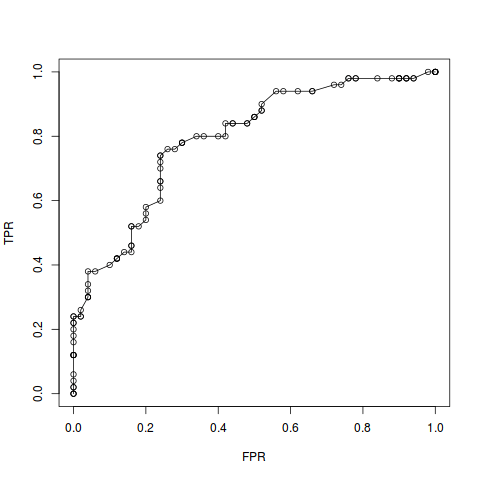Want to share your content on R-bloggers? click here if you have a blog, or here if you don't.

Unfortunately this was not taught in any of my statistics or data analysis classes at university (wtf it so needs to be :scream_cat:). So it took me some until I learned that the AUC has a nice probabilistic meaning.

## What’s AUC anyway?

Consider:

1. A dataset : , where
• is a vector of features collected for the th subject,
• is the th subject’s label (binary outcome variable of interest, like a disease status, class membership, or whatever binary label).
2. A classification algorithm (like logistic regression, SVM, deep neural net, or whatever you like), trained on , that assigns a score (or probability) to any new observation signifying how likely its label is .

Then:

1. A decision threshold (or operating point) can be chosen to assign a class label ( or ) to based on the value of . The chosen threshold determines the balance between how many false positives and false negatives will result from this classification.
2. Plotting the true positive rate (TPR) against the false positive rate (FPR) as the operating point changes from its minimum to its maximum value yields the receiver operating characteristic (ROC) curve. Check the confusion matrix if you are not sure what TPR and FPR refer to.
3. The area under the ROC curve, or AUC, is used as a measure of classifier performance.

Here is some R code for clarification (not even using tidyverse :stuck_out_tongue:):

# load some data, fit a logistic regression classifier
data(iris)
versicolor_virginica <- iris[iris$Species != "setosa", ] logistic_reg_fit <- glm(Species ~ Sepal.Width + Sepal.Length, data = versicolor_virginica, family = "binomial") y <- ifelse(versicolor_virginica$Species == "versicolor", 0, 1)
y_pred <- logistic_reg_fit\$fitted.values

# get TPR and FPR at different values of the decision threshold
threshold <- seq(0, 1, length = 100)
FPR <- sapply(threshold,
function(thresh) {
sum(y_pred >= thresh & y != 1) / sum(y != 1)
})
TPR <- sapply(threshold,
function(thresh) {
sum(y_pred >= thresh & y == 1) / sum(y == 1)
})

# plot an ROC curve
plot(FPR, TPR)
lines(FPR, TPR)


A rather ugly ROC curve emerges:The area under the ROC curve, or AUC, seem like a nice heuristic to evaluate and compare the overall performance of classification models independent of the exact decision threshold chosen. But there’s more to it.

## Probabilistic interpretation

As above, assume that we are looking at a dataset where we want to distinguish data points of type 0 from those of type 1. Consider a classification algorithm that assigns to a random observation a score (or probability) signifying membership in class 1. If the final classification between class 1 and class 0 is determined by a decision threshold , then the true positive rate (a.k.a. sensitivity or recall) can be written as a conditional probability